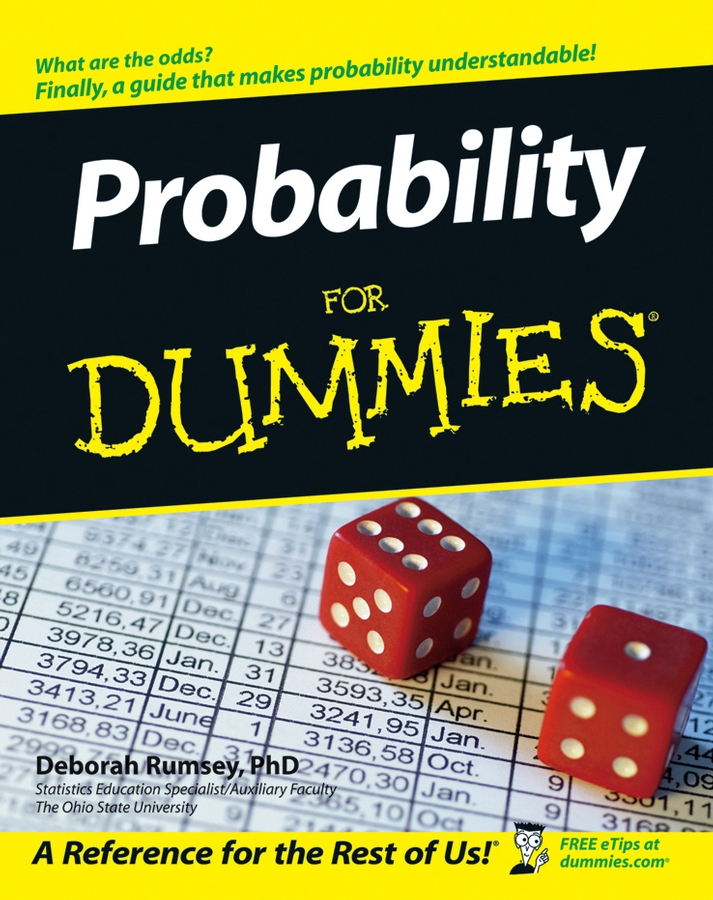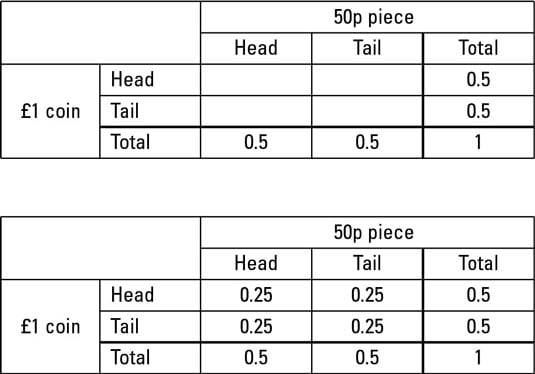##### Probability For DummiesA probability table is a way of representing probabilities. The following probability table looks at the events ‘throwing a head on a fair 50p piece’ and ‘throwing a head on a fair £1 coin.’You use the total probability of each event to work out the probability of each pair of events. Here’s how to draw your probability table:

1. Count how many possible outcomes the first event has.

Your table needs that many columns, plus another two.

2. Count how many possible outcomes the second event has.

Your table needs that many rows, plus another two.

3. Draw a table with the appropriate number of rows and columns.

4. Label the columns.

Leave the first column blank and then list the outcomes for the first event, followed by ‘total’ in the final column.

5. Label the rows.

Leave the first row blank and then list the outcomes for the second event, followed by ‘total’ in the final row.

6. The total of all the probabilities has to be one, so put 1 in the ‘total/total’ cell in the bottom right.

7. Find the probability of one of the events and write it in the ‘total’ box for that row or column.

Do the same for the other event.

8. Find the probability of a combined event.

If you already know this probability, write it in the right box. If not, times the two ‘total’ numbers you worked out in Step 7 and write the answer in the box in the same row and column as both.

9. Fill in the remaining boxes.

The cells in any row or column have to add up to the ‘total’ at the end or bottom of it.

Now you can look up the probability of any event by finding the right row and column.